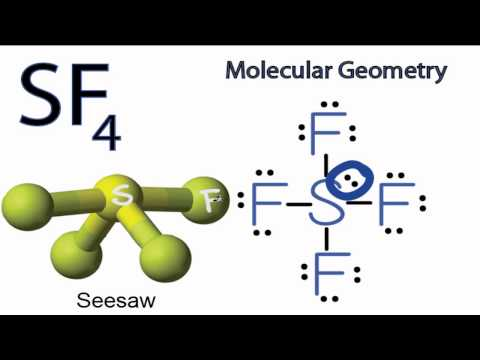Is the molecule SF_4 polar or non polar?

Apr 22, 2014

The easiest way to determine if a molecule is polar or nonpolar is to draw its Lewis Structure and, if necessary, check its molecular geometry.

Explanation:

If there is an odd number of lone pairs of electrons around the central atom, the molecule is polar.

If there is an even number of lone pairs, you must check the VSEPR structure to decide.

In your example of ${\text{SF}}_{4}$, the Lewis Structure would look like this:You can see that there is a lone electron pair around the sulfur atom and thus, the molecule is polar! :)

Here's why the "odd number of lone pairs" method works.

In VSEPR theory, the lone pair forces the molecular geometry of ${\text{SF}}_{4}$ into a see-saw shape.Two of the S-F bonds are pointing away from each other, and their bond dipoles cancel.

But the other two $\text{S-F}$ dipoles are pointing "down".

Their bond dipoles do not cancel, so the molecule is polar.

On the other hand, ${\text{XeF}}_{4}$ has two lone pairs on $\text{Xe}$.

This is an even number, so you have to check the shape of the molecule.Here, the $\text{Xe-F}$ bond dipoles cancel each other, so the molecule is nonpolar.

Hope this helps!